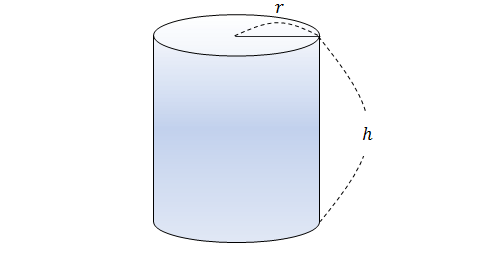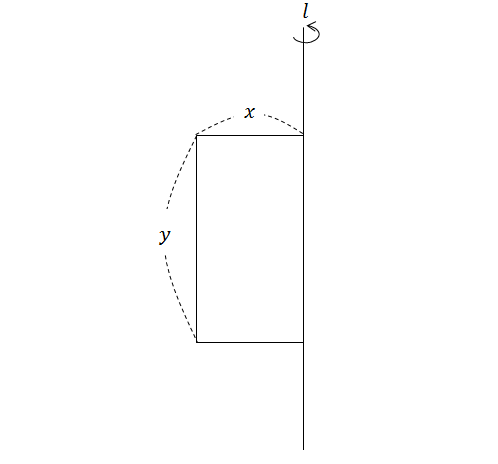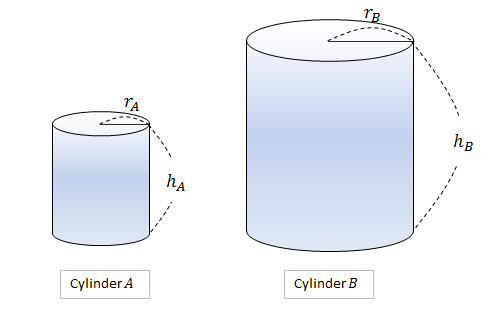Geometry

# Surface Area - Cylinder

What is the surface area of a cylinder with radius $$4$$ and height $$6$$?The above diagram shows a cylinder with height $$h=9.$$ If the surface area of the cylinder is $$44\pi,$$ what is the radius $$r?$$

Note: The above figure is not drawn to scale.The above diagram shows a rectangle with $$x=4$$ and $$y=8.$$ If the rectangle is rotated $$360^\circ$$ around line $$l,$$ what is the surface area of the resulting body?

Note: The above figure is not drawn to scale.The diagram above shows two cylinders with $$r_A=2, h_A=4, r_B=3,$$ and $$h_B=7.$$ If $$S_A$$ and $$S_B$$ are the surface areas of cylinders A and B, respectively, what is the value of $$\frac{S_A}{S_B}?$$

Note: The above figures are not drawn to scale.

A cylinder has a total surface area of $$136\pi$$. If it has a radius of $$4$$, what is its height?

×